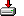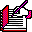#array.sas```%macro array (

array
, items
, sep = %str( )
, scope = RESOLVE
, lets =
, locals =

);

%* Richard A. DeVenezia
%* 031119 - Revised as [array], added scope, lets and locals
%* 931109 - Initial coding as [makelist]
%*
%* create a macro array from a delimited list of items
%*
%*          value is
%*          ----------------------------------------------------------------
%* array  - macro array name, prefix of numbered macro variables that
%*          will contain the item values
%* items  - original list of items, separated by sep
%* sep    - separator between items of items (incoming)
%* scope  - scope of macro array variables.
%*          GLOBAL, INHERIT, RESOLVE
%*          GLOBAL - avoid if possible
%*          INHERIT - the invoker _must_ ensure the variables
%*            <&array>_size <&array>_1 ... <&array>_n exist
%*            prior to invoking %array.
%*            Why? because a macro is not allowed to create a macro variable
%*            in a local scope above its own.
%*            (It may however, access any macro variables in scope above itself)
%*            If this macro implicitly 'creates' a macro variable, it will
%*            be destroyed when the macro ends, and thus will not be available
%*            to invoker.
%*          RESOLVE - macro var named in lets will receive a quoted macro
%*            statement which is a series of %lets.  The invoker is responsible
%*            for unquoting the statement to get macro vars in its scope.
%* lets   - name of macro var existing in invokers scope.
%*          upon return, the invoker should unquote the value
%*          to cause the macro array variables to be assigned.
%* locals - name of macro var existing in invokers scope.
%*          upon return, the invoker should resolve this variable in a
%*          %local statement to ensure the variables in the lets variable
%*          will not accidently overwrite an existing macro variable in scope
%*          higher than invoker. (See examples at bottom)
%*;

%if (&array. =) %then %do;
%put ERROR: array name is missing;
%goto EndMacro;
%end;

%let scope = %upcase(&scope);

%if 0 = %index (|GLOBAL|INHERIT|RESOLVE|, |&SCOPE.|) %then %do;
%put ERROR: scope = &scope is unknown;
%goto EndMacro;
%end;

%if (&scope = RESOLVE) and (&lets = ) %then %do;
%put ERROR: scope=&scope requires an lets=<macro-var>;
%goto EndMacro;
%end;

%let lets = %upcase(&lets);

%if (&scope = RESOLVE) and (&lets = LETS) %then %do;
%put ERROR: lets= can not be LETS, try lets=_let;
%goto EndMacro;
%end;

%let locals = %upcase(&locals);
%if (&locals=LOCALS) %then %do;
%put ERROR: locals= can not be LOCALS, try locals=_local;
%goto EndMacro;
%end;

%local item N local;

%let N=1;

%if  (&scope = GLOBAL) %then
%global &array._size;

%if (&scope = RESOLVE) %then
%let &lets = ;

%if (&locals ^= ) %then
%let &locals = &array._size;

%let item = %scan(&items,&N,%quote(&sep));

%do %while (&item ^= );

%if &scope = GLOBAL %then
%global &array.&N;

%if (&scope = GLOBAL) or (&scope = INHERIT) %then
%let &array.&N = &item;
%else
%let &lets = %nrquote(&&&lets)%nrstr(%let )&array.&N=&item%str(;);

%if (&locals ^= ) %then
%let &locals = &&&locals &array&N;

%let N = %eval(&N + 1);
%let item = %scan(&items,&N,%quote(&sep));

%end;

%let N = %eval(&N - 1);

%if (&scope = GLOBAL) or (&scope = INHERIT) %then
%let &array._size = &N;
%else
%let &lets = %nrquote(&&&lets)%nrstr(%let )&array._size=&N%str(;);

%EndMacro:

%mend;```

Sample code

```/*
%array (bob, a b c d e, scope=GLOBAL)
data _null_;
do i = 1 to &bob_size;
name = "BOB"||put(i,3.-L);
value = symget (name);
put i= name= value=;
end;
run;

%* mis-application of INHERIT
%* macro vars that will be the macro array should exist in invokers scope
%* prior to using %array;
%array (x, a b c d e, scope=INHERIT)
%put &x_size;

%* mis-application of INHERIT;
%* dangerous because only some vars are available as GLOBAL, and others not
%let y_size=;
%let y1=;
%let y3=;
%array (y, a b c d e, scope=INHERIT)
%put &y_size;
%put &y1;
%put &y2;
%put &y3;
%put &y4;
%put &y5;

%* mis-application of RESOLVE, init should exist prior to invocation;
%array (z, a b c d e, scope=RESOLVE, lets=init);
%put &lets;

%* proper application of RESOLVE, init exists prior to invocation;
%let lets=;
%array (z, a b c d e, scope=RESOLVE, lets=init);
%put &init;
%unquote(&init)
%put &z_size;
%put &z1;
%put &z2;
%put &z3;
%put &z4;
%put &z5;

%* proper application of RESOLVE, _let and _local exist prior to invocation;```
```%macro foo;
%local _let _local array;

%let array=xyz;

%array (&array, a b c d e, scope=RESOLVE, lets=_let, locals=_local);

%local &_local;

%unquote (&_let)

%do i = 1 %to &&&array._size;
%put &array&i=&&&array.&i;
%end;
%mend;
%foo
*/```